Homework Help Question & Answers

# A 3.10-g sample of lead nitrate, Pb(NO3)2, molar mass = 331 g/mol, is heated in an...

A 3.10-g sample of lead nitrate, Pb(NO3)2, molar mass = 331 g/mol, is heated in an evacuated cylinder with a volume of 2.00 L. The salt decomposes when heated, the unbalanced equation for the decomposition reaction is shown below: Pb(NO3)2(s) → PbO(s) + NO2(g) + O2(g) Assuming complete decomposition, what is the pressure in the cylinder after decomposition and cooling to a temperature of 303 K? Assume the PbO(s) takes up negligible volume.

#### Homework Answers

Answer #1

Molar mass of Pb(NO3)2 = 331 g/mol

mass of Pb(NO3)2 = 3.1 g
mol of Pb(NO3)2 = (mass)/(molar mass)
= 3.1/331
= 9.37*10^-3 mol

Balanced chemical equation is:
2Pb(NO3)2 ---> 2 PbO + 4 NO2 + O2

According to balanced equation
mol of gas formed = (5/2)* moles of Pb(NO3)2
= (5/2)* 9.37*10^-3
= 2.34*10^-2 mol

Given:
V = 2.0 L
n = 0.0234 mol
T = 303.0 K

use:
P * V = n*R*T
P * 2 L = 0.0234 mol* 0.08206 atm.L/mol.K * 303 K
P = 0.2909 atm
Answer: 0.291 atm

answered by: ANURANJAN SARSAM
Know the answer?
Your Answer:

#### Post as a guest

Your Name:

What's your source?

#### Earn Coin

Coins can be redeemed for fabulous gifts.

Not the answer you're looking for? Ask your own homework help question. Our experts will answer your question WITHIN MINUTES for Free.
Similar Homework Help Questions
• ### For the reaction 2 KI + Pb(NO), — Pol, + 2 KNO, how many grams of lead(II) nitrate, Pb(NO3)2, are needed to react...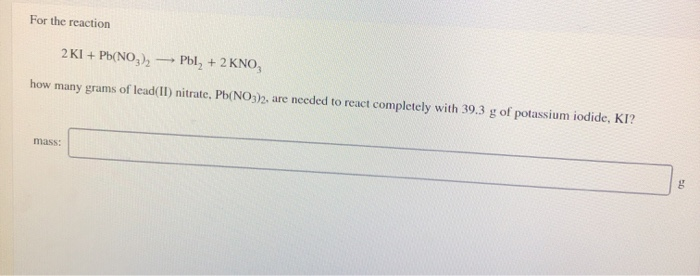For the reaction 2 KI + Pb(NO), — Pol, + 2 KNO, how many grams of lead(II) nitrate, Pb(NO3)2, are needed to react completely with 39.3 g of potassium iodide, KI? mass: 2 KI + Pb(NO3)2 Pol, + 2 KNO how many grams of lead(II) nitrate, Pb(NO3)2, are needed to react completely with 39.3 g of potassium iodide, KI? mass: Attem Small quantities of oxygen can be prepared in the laboratory by heating potassium chlorate, KCIO,(s). The equation for the...

• ### Potassium iodide reacts with lead (ii) nitrate in the following precipitation reaction: 2KI (aq) + Pb(NO3)2...

Potassium iodide reacts with lead (ii) nitrate in the following precipitation reaction: 2KI (aq) + Pb(NO3)2 (aq)---> 2KNO3 (aq) + PbI2 (s) What minimum volume of 0.200 M potassium iodide solution is required to completely precipitate all the lead in 155.0 mL of a 0.122 M lead (ii) nitrate solution?

• ### A solution of NaCl(aq) is added slowly to a solution of lead nitrate, Pb(NO3)2(aq) Pb ( NO 3 ) 2 ( aq ) , until no furth...

A solution of NaCl(aq) is added slowly to a solution of lead nitrate, Pb(NO3)2(aq) Pb ( NO 3 ) 2 ( aq ) , until no further precipitation occurs. The precipitate is collected by filtration, dried, and weighed. A total of 10.47 g PbCl2(s) 10.47 g PbCl 2 ( s ) is obtained from 200.0 mL 200.0 mL of the original solution. Calculate the molarity of the Pb(NO3)2(aq) Pb ( NO 3 ) 2 ( aq ) solution. concentration: M

• ### Part a please Question 3 (30 pts) Lead nitrate (Pb(NO3)2) is a toxic compound used in...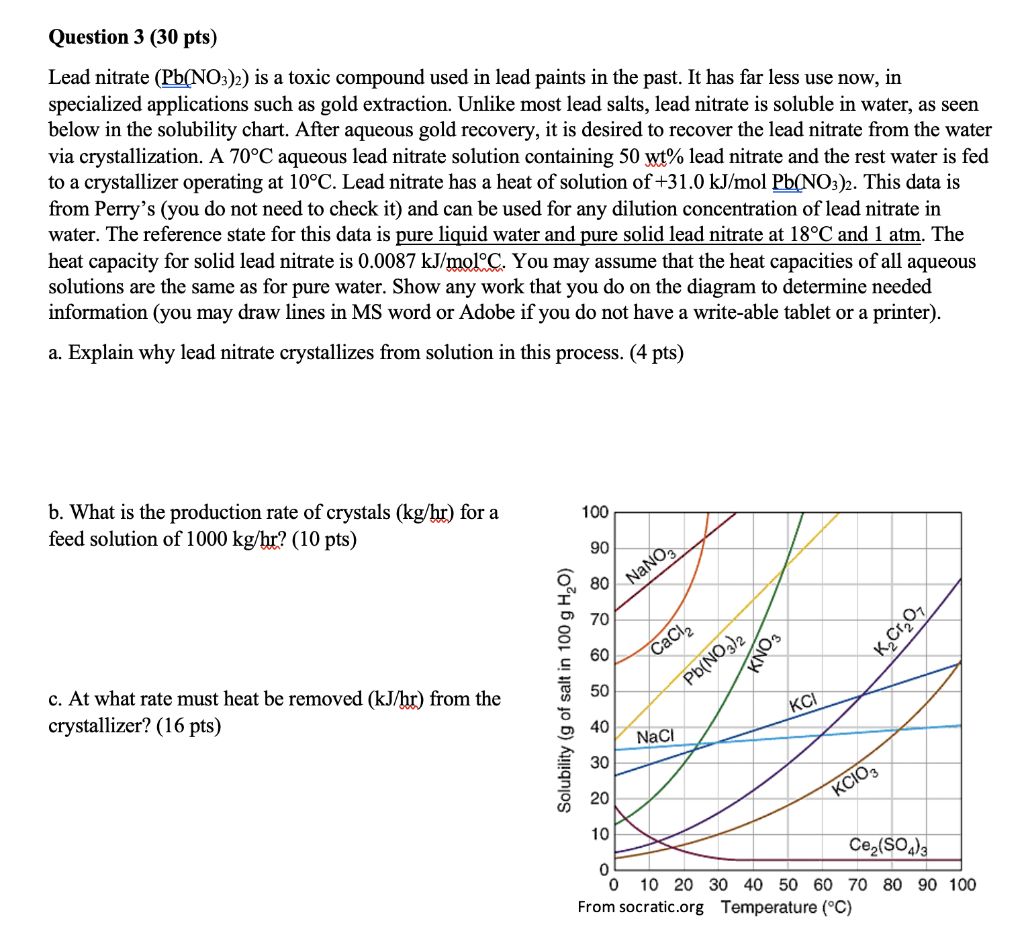Part a please Question 3 (30 pts) Lead nitrate (Pb(NO3)2) is a toxic compound used in lead paints in the past. It has far less use now, in specialized applications such as gold extraction. Unlike most lead salts, lead nitrate is soluble in water, as seen below in the solubility chart. After aqueous gold recovery, it is desired to recover the lead nitrate from the water via crystallization. A 70°C aqueous lead nitrate solution containing 50 wt% lead nitrate and...

• ### A solution of NaCl(aq) is added slowly to a solution of lead nitrate, Pb(NO3)2(aq), until no further precipitation occur...

A solution of NaCl(aq) is added slowly to a solution of lead nitrate, Pb(NO3)2(aq), until no further precipitation occurs. The precipitate is collected by filtration, dried, and weighed. A total of 15.59 g PbCl2(s) is obtained from 200.0 mL of the original solution. Calculate the molarity of the Pb(NO3)2(aq) solution. concentration: M

• ### When an excess of lead(II) carbonate, PbCO3(s), reacts with 27.5 mL of 3.00M nitric acid, HNO3(aq), what mass of lead(II) nitrate,Pb(NO3)2(aq) will be formed

When an excess of lead(II) carbonate, PbCO3(s), reacts with 27.5 mL of 3.00M nitric acid, HNO3(aq), what mass of lead(II) nitrate,Pb(NO3)2(aq) will be formed?

• ### 1. A 75.0-mL sample of 0.200 M Lead(II) nitrate, Pb(NO3)2, is reacted with 75.0 mL of...

1. A 75.0-mL sample of 0.200 M Lead(II) nitrate, Pb(NO3)2, is reacted with 75.0 mL of 0.450 M KI solution and the following precipitation reaction occurs. Pb(NO3)2(aq) + 2KI(aq) → PbI2(s) + 2KNO3(aq) (a) Determine the limiting reactant. (b) How many grams of PbI2 will be formed if the yield is 100%? (c) What is the percent yield if 6.45 g of PbI2 were obtained? (2) K2Cr2O7(aq) + FeCl2(aq) → CrCl3(aq) + Fe(NO3)3(aq) (a) Determine the net ionic reactions and...

• ### 0/ 1 pts Question 2 During a certain titration, 35.0 mL of 0.175 M aqueous sulfuric...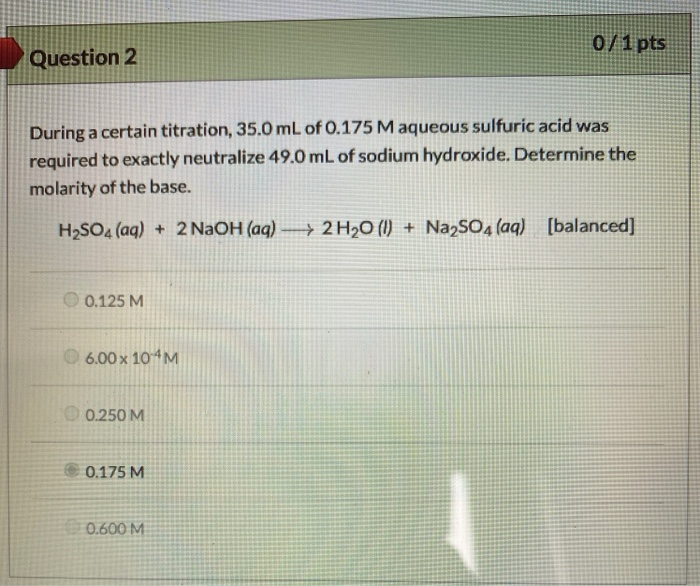0/ 1 pts Question 2 During a certain titration, 35.0 mL of 0.175 M aqueous sulfuric acid was required to exactly neutralize 49.0 mL of sodium hydroxide. Determine the molarity of the base. H2SO4 (aq) + 2NaOH(aq) + 2 H2O (0) + Na2SO4 (aq) [balanced] 0.125 M 6.00 x 10 M 0.250 M 0.175 M 0.600 M 2ct Question 6 0/1 pts Calculate the pressure exerted by 5.12 grams of chlorine gas in a 850 mL sealed flask at 27.0°C....

• ### LALUILLIE b. 3 NaOH(aq) + AlCl3(aq) - Al(OH)3(s) + 3 NaCl(aq) C. 2 H+ (aq) + Zn(s) - H2(g) + Zn + (aq) d. 2 HCl(aq)...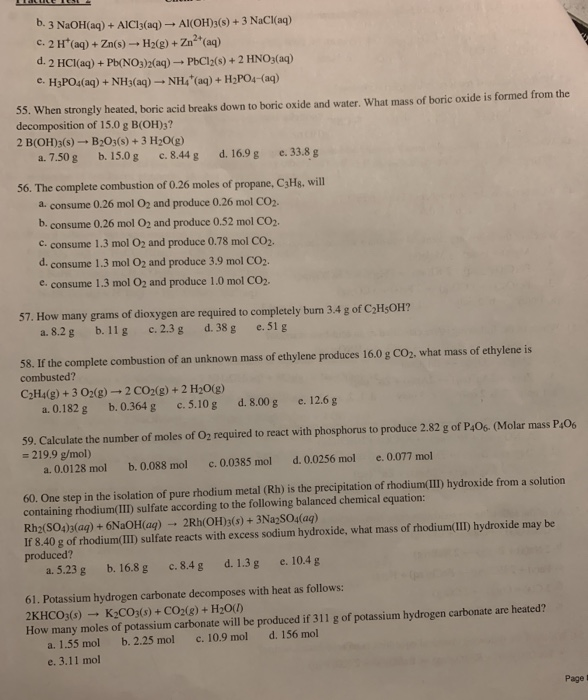LALUILLIE b. 3 NaOH(aq) + AlCl3(aq) - Al(OH)3(s) + 3 NaCl(aq) C. 2 H+ (aq) + Zn(s) - H2(g) + Zn + (aq) d. 2 HCl(aq) + Pb(NO3)2(aq) - PbCl2(s) + 2 HNO3(aq) e. H3PO4(aq) + NH3(aq) - NH(aq) + H2PO4-(aq) 33. When strongly heated, boric acid breaks down to boric oxide and water. What mass of boric oxide is formed from the decomposition of 15.0 g B(OH)3? 2 B(OH)3(s) - B2O3(s) + 3 H2O(g) a. 7.50 g b. 15.0...

• ### Exam Name MULTIPLE CHOICE. Choose the one alternative that best completes the statement or answers the...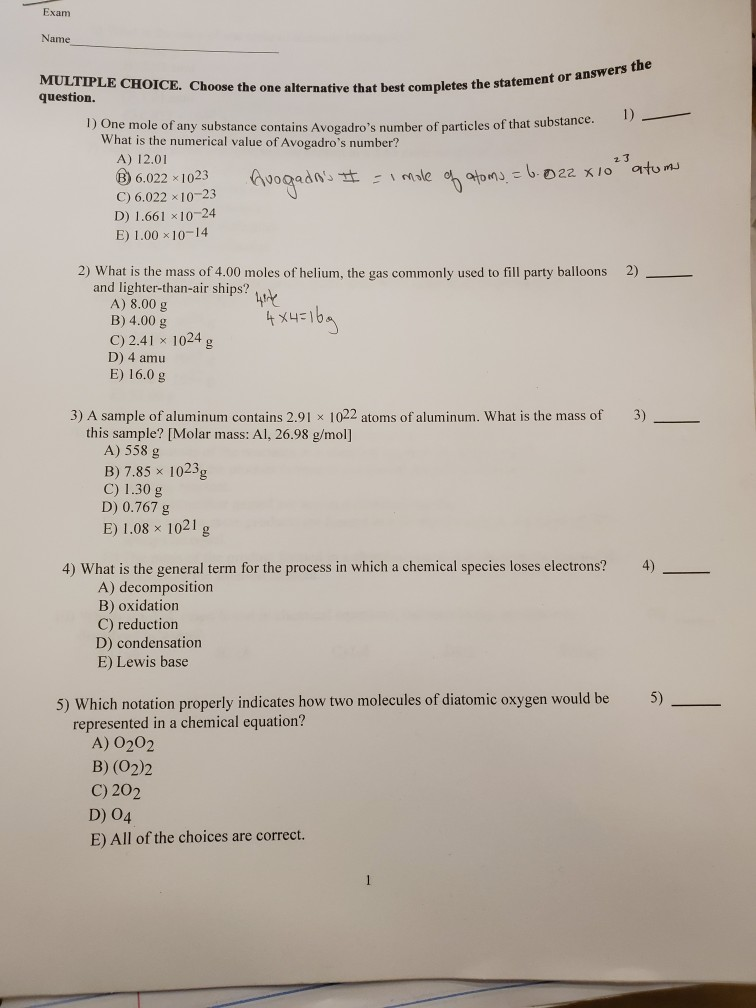Exam Name MULTIPLE CHOICE. Choose the one alternative that best completes the statement or answers the question. 1) 1) One mole of any substance contains Avogadro's number of particles of that substance. What is the numerical value of Avogadro's number? A) 12.01 23 Avagrans t Male aton 6022 x1o atums B) 6.022 x 1023 C) 6.022 x 10-23 D) 1.661 x10-24 E) 1.00 x10-14 2) What is the mass of 4.00 moles of helium, the gas commonly used to fill...

Free Homework App

Scan Your Homework
to Get Instant Free Answers
Need Online Homework Help?

Get Answers For Free
Most questions answered within 3 hours.